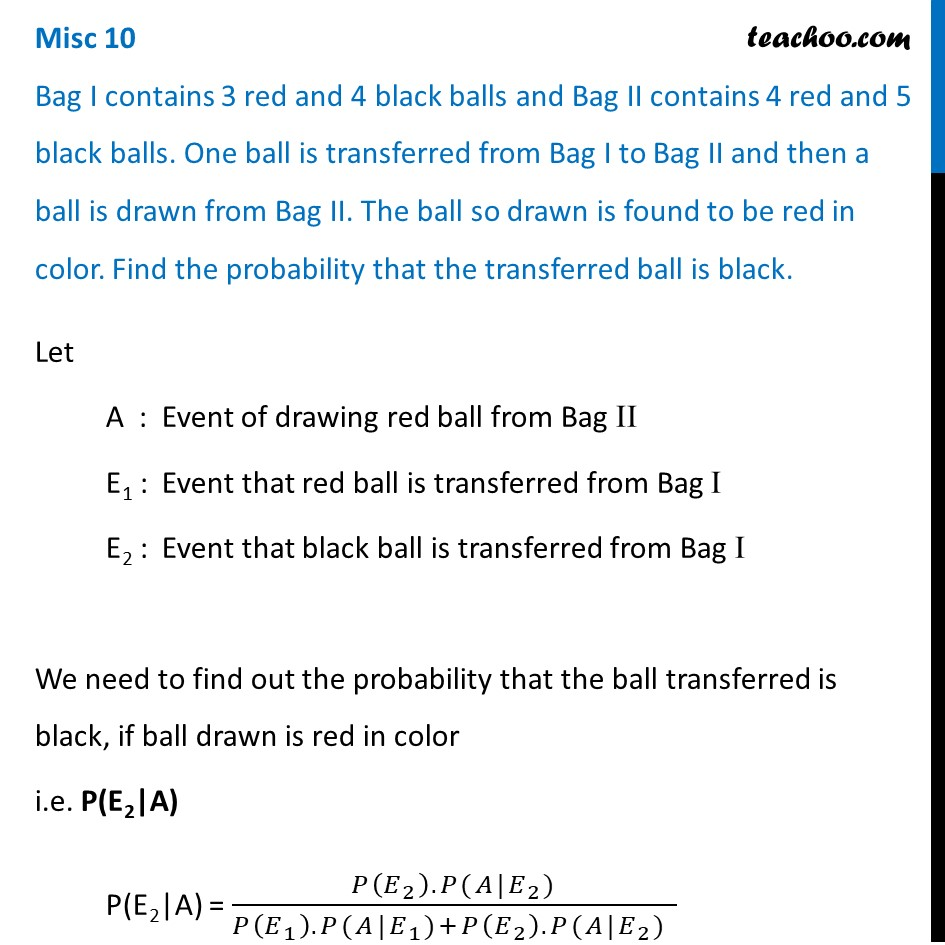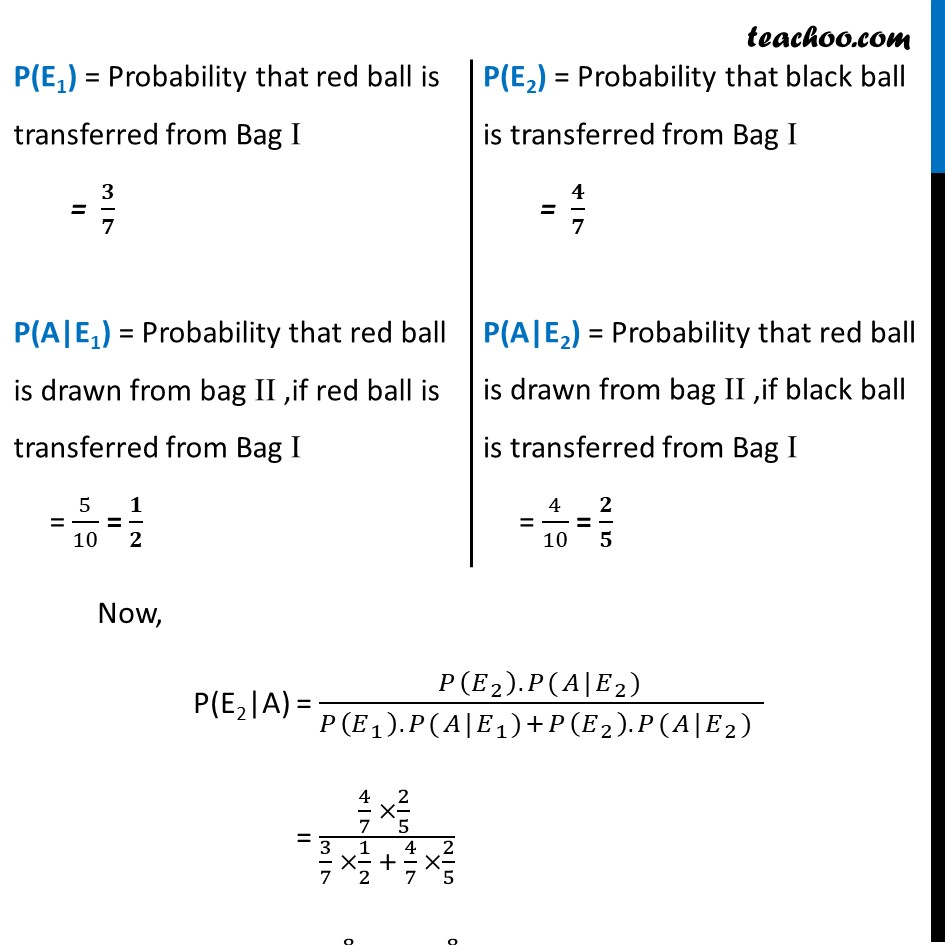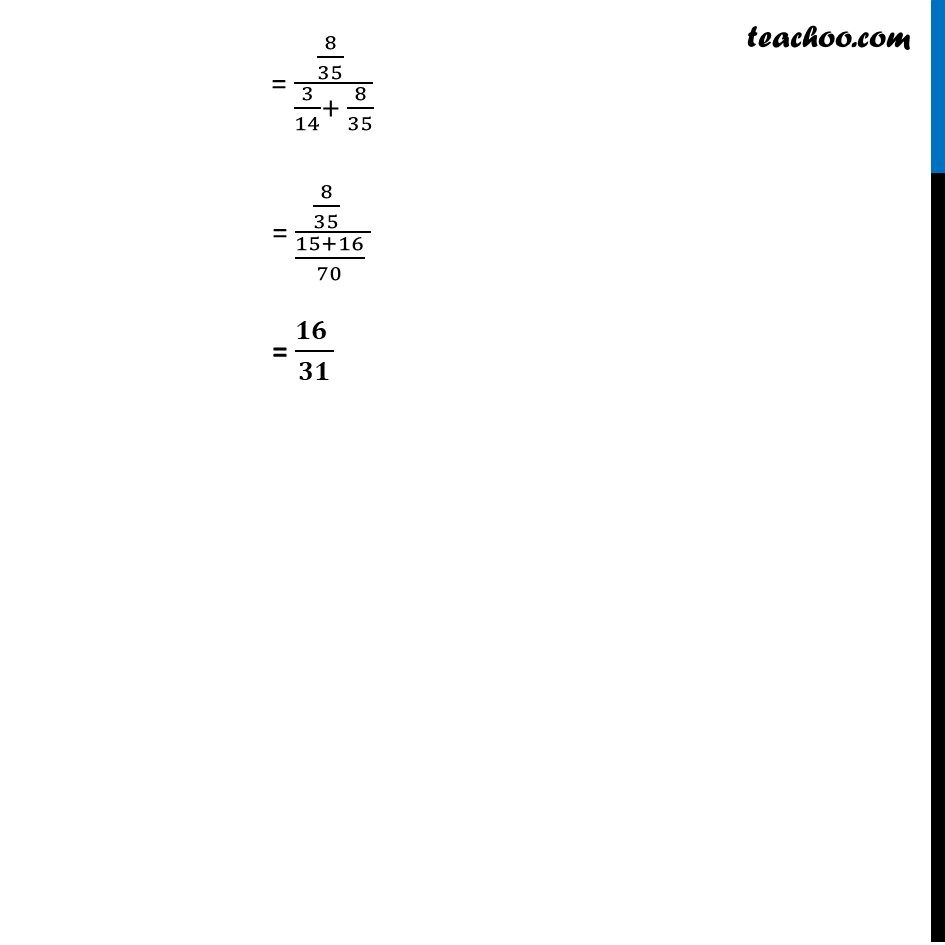Miscellaneous

Chapter 13 Class 12 Probability
Serial order wiseLearn in your speed, with individual attention - Teachoo Maths 1-on-1 Class

### Transcript

Misc 10 Bag I contains 3 red and 4 black balls and Bag II contains 4 red and 5 black balls. One ball is transferred from Bag I to Bag II and then a ball is drawn from Bag II. The ball so drawn is found to be red in color. Find the probability that the transferred ball is black. Let A : Event of drawing red ball from Bag II E1 : Event that red ball is transferred from Bag I E2 : Event that black ball is transferred from Bag I We need to find out the probability that the ball transferred is black, if ball drawn is red in color i.e. P(E2|A) P(E2|A) = (𝑃(𝐸_2 ).𝑃(𝐴|𝐸_2))/(𝑃(𝐸_1 ).𝑃(𝐴|𝐸_1)+𝑃(𝐸_2 ).𝑃(𝐴|𝐸_2) ) "P(E1)" = Probability that red ball is transferred from Bag I = 𝟑/𝟕 P(A|E1) = Probability that red ball is drawn from bag II ,if red ball is transferred from Bag I = 5/10 = 𝟏/𝟐 "P(E2)" = Probability that black ball is transferred from Bag I = 𝟒/𝟕 P(A|E2) = Probability that red ball is drawn from bag II ,if black ball is transferred from Bag I = 4/10 = 𝟐/𝟓 Now, P(E2|A) = (𝑃(𝐸_2 ).𝑃(𝐴|𝐸_2))/(𝑃(𝐸_1 ).𝑃(𝐴|𝐸_1)+𝑃(𝐸_2 ).𝑃(𝐴|𝐸_2) ) = (4/7 ×2/5 )/(3/7 ×1/2 + 4/7 ×2/5) = (8/35 )/(3/14+ 8/35) = (8/35 )/((15+16)/70 ) = (𝟏𝟔 )/𝟑𝟏 = (8/35 )/(3/14+ 8/35) = (8/35 )/((15+16)/70 ) = (𝟏𝟔 )/𝟑𝟏